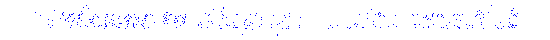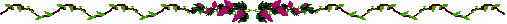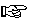Singapore DharmaNet Homepage
``````## About Time and Flat TimeHow do you think about time? Most people think of time as being like the frames in a cinema film except continuous. This idea of time is called "flat time" and is wrong. You can see how it is wrong by thinking about other ways of organising information. Information can easily be arranged in three independent ways in space. It can be arranged along a line, the lines can be arranged next to each other to make a plane and the planes can be stacked on top of each other to make a solid. Suppose you were trying to work out what a plane was like and, instead of arranging lines next to each other you just kept on making new arrangements along a single line. This long line might have the same content as a small, square plane but it would not be a plane. If you imagine time as a set of 3D images occuring one after the other you are making the same mistake as someone who imagines that a plane is just repeating sequences along a line. Once information has been arranged on a plane Pythagoras' theorem defines the separation of any two points in terms of the independent directions of arrangement (x and y). Eg: separation, h is given by: h^2 = x^2 + y^2 (Notice how the separation is less on a plane than it would have been if the information was arranged as repeating sequences on a line). Physical Time Physical theory defines time through the vehicle of Relativity theory. This physical time is a negative dimension and is experienced in a different way from positive dimensions. What defines a dimension is not only that it provides an independent way of arranging information but also that it is related to the other dimensions by Pythagoras' theorem. (Strictly, Pythagoras in differential form). The separation of two things in a 4 dimensional space-time, s, is given by: s^2 = x^2 + y^2 + z^2 - T^2 Now suppose you were sitting, looking at the world, it might come as a shock but everything you see has a separation of zero. It is no distance from where you are looking. Eg: 0 = x^2 + y^2 + z^2 - T^2 The things that you see are those positioned such that T^2 is equal to x^2 + y^2 + z^2, or, in other words you see those things where the distance in physical time equals the distance in space. So physical time is the thing that makes a whole out of all the parts of the image of the world in your brain. It is the thing that reduces the separation between the observation point and the observed to zero whilst keeping the observed thing in it's original position. (This sounds whacky but what is actually happening is that space disappears between the point of observation and the thing that is observed.) This is how we get the feeling of being a point eye looking out at things as if they are separate from us. Physical time creates a view of the present that is composed of information from the past. This is very different from "flat time" where the present is believed to be the same moment everywhere. Intriguingly, the gap between the universal present of "flat time" and the present of physical time represents events that have occurred but are as yet unknown. According to quantum theory these events should be in quantum superposition and only become fixed when they are observable. Phenomenal Time So much for physical time, the gobbler of space, but what about our sensation of events occurring and time passing? Perhaps time involves more than one extra dimension, perhaps there is another way of ordering information which might be called "phenomenal time". If phenomenal time is an independent dimension then it should be possible to observe several things at a point in space. The simplest example of this is when someone speaks, you hear the whole word, coming from their mouth, extended through time. Seeing that things are moving is a similar trick. Our experience of events suggests that there is indeed another time dimension.Return to Singapore DharmaNet